### 1. TDEmass – Mass inference method for tidal disruption events.

• #### Model Description

i. Model – slow circularization

TDEmass is a tool for interpretation of Tidal Disruption Event (TDE) observations. In TDEs, a supermassive black hole at the center of a galaxy tears apart an ordinary star; the debris is placed on highly eccentric orbits and, in ways that remain controversial, ultimately produces a very bright flare. The spectrum of the optical/UV light in this flare is generally well described by a black body with temperature ~ few  10,000 K.

Using this tool, one can infer the mass of the black hole () and the mass of the star (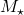) involved in a TDE by solving two non-linear algebraic equations derived in Ryu+2020 (https://ui.adsabs.harvard.edu/abs/2020arXiv200713765R/abstract) (Equations 9 and 10). These equations arise from a physical model for the optical/UV luminosity in which the energy for this light is generated by dissipation in shocks near the debris’ orbital apocenter. They may be written in the following form:

(1)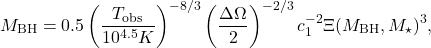(2)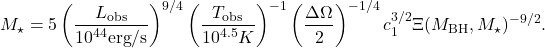where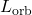refers to the observed peak UV/optical luminosity (in units of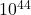erg/s) and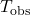are the observed temperature at peak luminosity (in units ofK).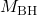areare in units of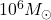and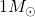, respectively. c_{1} and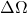are two unspecified parameters (see Section 2) below ).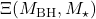is the ratio of the width of the debris’ specific energy distribution to its conventional estimate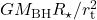where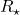is the stellar radius and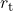is the order of magnitude estimate for the tidal radius, i.e.,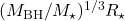(Ryu+2020, Arxiv 2001:03501).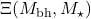is nonlinear, but analytic function ofand. Its nonlinearity is what requires numerical solutions of these equations.

ii. Two unspecified parametersandOur model includes two unspecified parameters,and.is the distance from the black hole at which a significant amount of energy is dissipated by shocks. Here,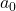is the apocenter distance for the orbit of the most tightly bound debris.is the solid angle. So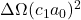corresponds to the surface area of the emitting region. Note thatis equivalent to what is called the “black body radius”. For fixed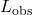and, largerleads to smallerand, but the dependence is weak. However,andare sensitive to. For fixedand,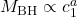with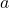= -(1.2-2) and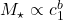with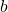= 0.8-1.5.

The quantities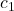andare coefficients poorly-determined by current theory; we recommend setting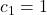and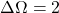(default values in the code).

• #### Code description

The code solves the equations using the following algorithm:

– Create a table ofandvalues on a logarithmic grid inandwithin ranges specified by mbh_range and mstar_range. There are 500 within each mass range.

– Locateandwithin this table and define the uncertainty inandby the uncertainty inand.
These uncertainties are called dLobs-, dLobs+, dTobs-, and dTobs+.

– Find the central values ofandwithin the ranges determined by the uncertainty inand(see find_centroid_range() in module.py for the definition of the central value)

– With the first guess, find new values ofandby using the two basic equations. Iterate to convergence. The initial values ofandare chosen to be the central values ofand. The routine embodying this step is solver1_LT().

– If the previous step fails to converge, use the 2-dimensional Newton-Raphson method to solve the equations.
The initial values ofandare the central values ofand. This routine is called solver2_LT().

The units in the code are:

– Luminosity(Lorb, Lobs_1, Lobs_2) = erg/s

– Temperature (Tobs, Tobs_1, Tobs_2) = K

– Black hole mass (mbh) =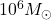(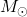: solar mass)

– Stellar mass (mstar) =https://github.com/taehoryu/TDE_mass_inference.git

To run, you first enter the directory “TDE_mass_inference”. In the directory, the code is run as

Python3 main.py

The code has three source dependencies : scipy, numpy and matplotlib

• #### Inputs and outputs

Refer to the detailed description here (Section 3 and 4)

• #### Issues/requests

E-mail address for issues/requests : udraeo@gmail.com